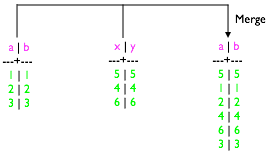# Example: Save Pig relations from/to Cassandra (deprecated)

How to merge the data from two CQL tables having simple primary keys using Pig.

Hadoop is deprecated for use with DataStax Enterprise. DSE Hadoop and BYOH (Bring Your Own Hadoop) are deprecated. Pig is also deprecated and will be removed when Hadoop is removed.

For Pig to access data in Cassandra, the target keyspace and table must already exist. Pig can save data from a Pig relation to a table in Cassandra and from a Cassandra table to a pig relation, but it cannot create the table. This example shows how to merge the data from two CQL tables having simple primary keys using Pig.A subsequent example shows how to merge data from CQL tables having compound primary keys into one CQL table using Pig.

## Procedure

1. Start cqlsh.
2. Using cqlsh, create and use a keyspace named, for example, cql3ks.
``````cqlsh> CREATE KEYSPACE cql3ks WITH replication =
{'class': 'SimpleStrategy', 'replication_factor': 1 };

cqlsh> USE cql3ks;``````
3. Create a two-column (a and b) Cassandra table named simple_table1 and another two-column (x and y) table named simple_table2. Insert data into the tables.
``````cqlsh:cql3ks> CREATE TABLE simple_table1 (a int PRIMARY KEY, b int);
cqlsh:cql3ks> CREATE TABLE simple_table2 (x int PRIMARY KEY, y int);
cqlsh:cql3ks> INSERT INTO simple_table1 (a,b) VALUES (1,1);
cqlsh:cql3ks> INSERT INTO simple_table1 (a,b) VALUES (2,2);
cqlsh:cql3ks> INSERT INTO simple_table1 (a,b) VALUES (3,3);
cqlsh:cql3ks> INSERT INTO simple_table2 (x, y) VALUES (4,4);
cqlsh:cql3ks> INSERT INTO simple_table2 (x, y) VALUES (5,5);
cqlsh:cql3ks> INSERT INTO simple_table2 (x, y) VALUES (6,6);``````
4. Using Pig, add logic to load the data (4, 5, 6) from the Cassandra simple_table2 table into a Pig relation.
``grunt> moretestvalues= LOAD 'cql://cql3ks/simple_table2/' USING CqlNativeStorage;``
5. Convert the simple_table2 table data to a tuple. The key column is a chararray, 'a'.
``````grunt> insertformat= FOREACH moretestvalues GENERATE
TOTUPLE(TOTUPLE('a',x)),TOTUPLE(y);``````
6. Save the relation to the Cassandra simple_table1 table.
``````grunt> STORE insertformat INTO
'cql://cql3ks/simple_table1?output_query=UPDATE+cql3ks.simple_table1+set+b+%3D+%3F'
USING CqlNativeStorage;``````

Pig uses a URL-encoded prepared statement to store the relation to Cassandra. The cql:// URL is followed by an output_query, which specifies which key should be used in the command. The rest of the arguments, the "?"s, for the prepared statement are filled in by the values related to that key in Pig.

7. On the cqlsh command line, check that the simple_table1 table now contains its original values plus the values from the simple_table2 table:
``cqlsh:cql3ks> SELECT * FROM simple_table1;``
``````

a | b
--+--
5 | 5
1 | 1
2 | 2
4 | 4
6 | 6
3 | 3``````